# Sobolev space

A space $W^l_p(\Omega)$ of functions $f=f(x)=f(x_1,\ldots,x_n)$ on a set $\Omega\subset\RR^n$ (usually open) such that the $p$-th power of the absolute value of $f$ and of its generalized derivatives (cf. Generalized derivative) up to and including order $l$ are integrable ($1\leq p\leq \infty$).

The norm of a function $f\in W^l_p(\Omega)$ is given by \begin{equation}\label{eq:1} \lVert f\rVert_{W^l_p(\Omega)}=\sum_{\lvert k\rvert\leq l} \lVert f^{(k)}\rVert_{L_p(\Omega)}. \end{equation} Here \begin{equation*} f^{(k)}=\frac{\partial^{\lvert k\rvert}f}{\partial x_1^{k_1}\cdots \partial x_n^{k_n}},\qquad f^{(0)}=f, \end{equation*} is the generalized partial derivative of $f$ of order $\lvert k\rvert=\sum_{j=1}^n k_j$, and \begin{equation*} \lVert \psi\rVert_{L_p(\Omega)} =\left( \int_\Omega \lvert\psi(x)\rvert^p\,dx \right)^{1/p} \qquad (1\leq p\leq \infty). \end{equation*}

When $p=\infty$, this norm is equal to the essential supremum: \begin{equation*} \lVert \psi\rVert_{L_\infty(\Omega)} =\operatorname*{ess sup}_{x\in\Omega}\lvert\psi(x)\rvert \qquad (p=\infty), \end{equation*} that is, to the greatest lower bound of the set of all $A$ for which $A<\lvert\psi(x)\rvert$ on a set of measure zero.

The space $W^l_p(\Omega)$ was defined and first applied in the theory of boundary value problems of mathematical physics by S.L. Sobolev (see , ).

Since its definition involves generalized derivatives rather than ordinary ones, it is complete, that is, it is a Banach space.

$W^l_p(\Omega)$ is considered in conjunction with the linear subspace $W^l_{pc}(\Omega)$ consisting of functions having partial derivatives of order $l$ that are uniformly continuous on $\Omega$. $W^l_{pc}(\Omega)$ has advantages over $W^l_p(\Omega)$, although it is not closed in the metric of $W^l_p(\Omega)$ and is not a complete space. However, for a wide class of domains (those with a Lipschitz boundary, see below) the space $W^l_{pc}(\Omega)$ is dense in $W^l_p(\Omega)$ for all $p$, $1\leq p<\infty$, that is, for such domains the space $W^l_p(\Omega)$ acquires a new property in addition to completeness, in that every function belonging to it can be arbitrarily well approximated in the metric of $W^l_p(\Omega)$ by functions from $W^l_{pc}(\Omega)$.

It is sometimes convenient to replace the expression \eqref{eq:1} for the norm of $f\in W^l_p(\Omega)$ by the following: \begin{equation}\label{eq:2} \lVert f\rVert^\prime_{W^l_p(\Omega)}=\left( \int_\Omega \sum_{\lvert k\rvert\leq l} \lvert f^{(k)}(x)\rvert^p \,dx \right)^{1/p} \qquad (1\leq p<\infty). \end{equation} The norm \eqref{eq:2} is equivalent to the norm \eqref{eq:1}, i.e. $c_1 \lVert f\rVert \leq \lVert f\rVert^\prime \leq c_2 \lVert f\rVert$, where $c_1, c_2>0$ do not depend on $f$. When $p=2$, \eqref{eq:2} is a Hilbert norm, and this fact is widely used in applications.

The boundaryof a bounded domainis said to be Lipschitz if for any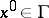there is a rectangular coordinate system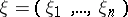with origin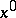so that the box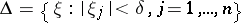is such that the intersection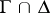is described by a function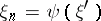, withwhich satisfies on(the projection ofonto the plane) the Lipschitz condition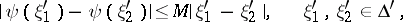where the constantdoes not depend on the points, and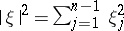. All smooth and many piecewise-smooth boundaries are Lipschitz boundaries.

For a domain with a Lipschitz boundary, (1) is equivalent to the following:where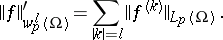One can consider more general anisotropic spaces (classes)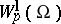, whereis a positive vector (see Imbedding theorems). For every such vectorone can define, effectively and to a known extent exhaustively, a class of domainswith the property that if, then any function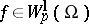can be extended towithin the same class. More precisely, it is possible to define a function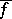onwith the properties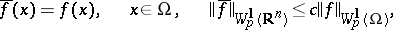wheredoes not depend on(see ).

In virtue of this property, inequalities of the type found in imbedding theorems for functions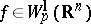automatically carry over to functions,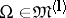.

For vectors, the domainshave Lipschitz boundaries, and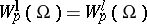.

The investigation of the spaces (classes)() is based on special integral representations for functions belonging to these classes. The first such representation was obtained (see , ) for an isotropic spaceof a domain, star-shaped with respect to some sphere. For the further development of this method see, for example, .

The classes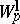and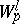can be generalized to the case of fractional, or vectors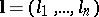with fractional components.

The space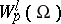can also be defined for negative integers. Its elements are usually generalized functions, that is, linear functionals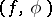on infinitely-differentiable functionswith compact support in.

By definition, a generalized functionbelongs to the class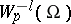() ifis finite, where the supremum is taken over all functionswith norm at most one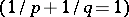. The functionsform the space adjoint to the Banach space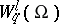.

How to Cite This Entry:
Sobolev space. Encyclopedia of Mathematics. URL: http://encyclopediaofmath.org/index.php?title=Sobolev_space&oldid=25915
This article was adapted from an original article by S.M. Nikol'skii (originator), which appeared in Encyclopedia of Mathematics - ISBN 1402006098. See original article